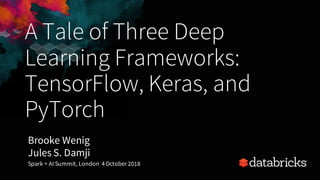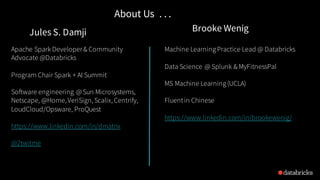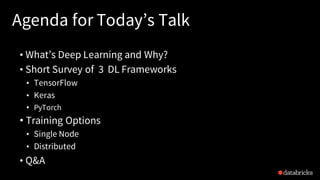Successfully reported this slideshow.

# A Tale of Three Deep Learning Frameworks: TensorFlow, Keras, & PyTorch with Brooke Wenig and Jules Damji×

1 of 39 Ad
1 of 39 Ad

# A Tale of Three Deep Learning Frameworks: TensorFlow, Keras, & PyTorch with Brooke Wenig and Jules Damji

We all know what they say – the bigger the data, the better. But when the data gets really big, how do you mine it and what deep learning framework to use? This talk will survey, with a developer’s perspective, three of the most popular deep learning frameworks—TensorFlow, Keras, and PyTorch—as well as when to use their distributed implementations.

We’ll compare code samples from each framework and discuss their integration with distributed computing engines such as Apache Spark (which can handle massive amounts of data) as well as help you answer questions such as:

As a developer how do I pick the right deep learning framework?

Do I want to develop my own model or should I employ an existing one?

How do I strike a trade-off between productivity and control through low-level APIs?

What language should I choose?

In this session, we will explore how to build a deep learning application with Tensorflow, Keras, or PyTorch in under 30 minutes. After this session, you will walk away with the confidence to evaluate which framework is best for you.

We all know what they say – the bigger the data, the better. But when the data gets really big, how do you mine it and what deep learning framework to use? This talk will survey, with a developer’s perspective, three of the most popular deep learning frameworks—TensorFlow, Keras, and PyTorch—as well as when to use their distributed implementations.

We’ll compare code samples from each framework and discuss their integration with distributed computing engines such as Apache Spark (which can handle massive amounts of data) as well as help you answer questions such as:

As a developer how do I pick the right deep learning framework?

Do I want to develop my own model or should I employ an existing one?

How do I strike a trade-off between productivity and control through low-level APIs?

What language should I choose?

In this session, we will explore how to build a deep learning application with Tensorflow, Keras, or PyTorch in under 30 minutes. After this session, you will walk away with the confidence to evaluate which framework is best for you.

### A Tale of Three Deep Learning Frameworks: TensorFlow, Keras, & PyTorch with Brooke Wenig and Jules Damji

1. 1. A Tale of Three Deep Learning Frameworks: TensorFlow, Keras, and PyTorch Brooke Wenig Jules S. Damji Spark + AI Summit, London 4October 2018
2. 2. About Us . . . Machine LearningPractice Lead @ Databricks Data Science @ Splunk & MyFitnessPal MS Machine Learning(UCLA) Fluentin Chinese https://www.linkedin.com/in/brookewenig/ Brooke WenigJules S. Damji Apache Spark Developer& Community Advocate @Databricks Program Chair Spark + AI Summit Software engineering @Sun Microsystems, Netscape, @Home, VeriSign, Scalix, Centrify, LoudCloud/Opsware, ProQuest https://www.linkedin.com/in/dmatrix @2twitme
3. 3. Agenda for Today’s Talk • What’s Deep Learning and Why? • Short Survey of 3 DL Frameworks • TensorFlow • Keras • PyTorch • Training Options • Single Node • Distributed • Q&A
4. 4. What is Deep Learning? “Composing representations of data in a hierarchical manner”
5. 5. Why Deep Learning?
6. 6. Applications Source : MIT
7. 7. Zoo of DL Frameworks: Which One? DPL
8. 8. Survey of Three Deep Learning Frameworks
9. 9. What’s TensorFlow? • Open source from Google, 2015 • Current v1.12 API • 2.0 Coming Soon... :) • Declarative Toolkit • Fast: Backend C/C++ • Data flow graphs • Nodes are functions/operators • Edges are input or data (tensors) • Lazy execution • Eager execution (1.7)
10. 10. TensorFlow Key API Concepts • Constants • Variables • Placeholders • Operations • Sessions • Tensors • Graphs • x = tf.constants (42, name= ‘x’) w = tf.Variable(1.34,name=’w’) input= tf.Placeholder(“float”) c = tf.add(x, w); m = tf.matmul(a, b) ... with tf.Session([URI]) as sess: 1, [1, 2], [[2, 3], [4, 5]] ... g = tf.Graph(“my_graph”) withg.as_default(): c = tf.add(x,w) m= tf.matmul(a, b)
11. 11. TensorFlow Code import tensorflow as tf a = tf.placeholder(tf.float32, shape=(2,1)) b = tf.placeholder(tf.float32, shape=(1,2)) c = tf.matmul(a, b) sess = tf.Session() print(sess.run(c, {a: [,], b:[[3,4]]})) [[3. 4.] [6. 8.]] Create a TF Session Run the session, with input parameters for place holders ‘a’ & ’b’ ‘c’ as an operation won’t to run until sess.run() Lazily evaluated. TF matmul matrix operation TF session output Create TF placeholder types, a & b Define their input shape as tensors
12. 12. TensorFlow Code: MNIST import tensorflow as tf from tensorflow.examples.tutorials.mnist import input_data mnist = input_data.read_data_sets("MNIST_data/", one_hot=True) # Create the model x = tf.placeholder(tf.float32, [None, 784]) W = tf.Variable(tf.zeros([784, 10])) b = tf.Variable(tf.zeros()) y = tf.matmul(x, W) + b y_ = tf.placeholder(tf.int64, [None]) ... # Define loss and optimizer cross_entropy = tf.losses.sparse_softmax_cross_entropy(labels=y_, logits=y) train_step = tf.train.GradientDescentOptimizer(0.5).minimize(cross_entropy) #create session, train, and evaluate sess = tf.InteractiveSession() tf.global_variables_initializer().run() # Train for _ in range(1000): batch_xs, batch_ys = mnist.train.next_batch(100) sess.run(train_step, feed_dict={x: batch_xs, y_: batch_ys}) # Test trained model correct_prediction = tf.equal(tf.argmax(y, 1), y_) accuracy = tf.reduce_mean(tf.cast(correct_prediction, tf.float32)) ... print(sess.run(accuracy, feed_dict={ x: mnist.test.images, y_: mnist.test.labels })) https://github.com/tensorflow/tensorflow/blob/master/tensorflow/examples/tutorials/mnist/mnist_softmax.py Use tf input_data modules for MNIST TF placeholders & variables Define our model TF variable for predicted value y’ Define our loss function: cross_entropy Use Gradient Descent Optimizer Train or evaluate the model
13. 13. TensorFlow Programming Stack CPU GPU Android iOS …TPU Use canned estimators Build models Keras Models
14. 14. Why TensorFlow: Community AF AF • 105K+ stars! • 11+M downloads • Popular open-source code • TensorFlow Hub & Blog ○ Code Examples & Tutorials! ○ Learn + share from others
15. 15. Why TensorFlow: Tools AF AF Deploy + Serve Models TFX Visualize Tensors flow
16. 16. TensorFlow: We Get it … So What? • Steep learning curve, but powerful!! • Low-level APIs, butoffers control!! • Expert in Machine Learning, justlearn!! • Yet, high-level Estimators help, you bet!! • Yeah, TensorFlow 2.0, ease-of-use,eagerexecution! • Better, Keras integration helps, indeed!!
17. 17. What’s Keras? • Open source Python Library APIs for Deep Learning • Current v2.2.2 APIs François Chollet (Google) • APIs : with TensorFlow, CNTK and Theano Backends • Easy to UseHigh-Level DeclarativeAPIs! • Build layers – Great for Neural Network Applications – CNN – RNN & LSTM • Fast Experimentation,Modular & Extensible!
18. 18. Keras Programming Stack CPU GPU Android iOS …TPU Use canned estimators Specific Runtime Backend Impl models Python Keras API TF-Keras Theano-Keras CNTK TensorFlow Workflow .....
19. 19. Why Keras? • Focuses on Developer Experience • Popular & Broader Community • Supports multiple backends • Modularity • Sequential • Functional model = Sequential() model.add(Dense(32, input_dim=784)) model.add(Activation('relu')) model.add(Dense, 32, activation=’softmax’) ...
20. 20. Keras Code: MNIST from keras import models from keras import layers mnist = tf.keras.datasets.mnist (train_images, train_labels),(test_images, test_labels)= prepare_data(mnist.load_data()) network= models.Sequential() network.add(layers.Dense(512, activation=‘relu’, input_shape(28 * 28,))) network.add(layers.Dense(10, activation=‘softmax’)) network.compile(optimizer=’rmsprop', loss='categorical_crossentropy', metrics=['accuracy']) network.fit(train_images, train_labels, epochs=5, batch_size=128 ) results = network.evaluate(test_images, test_labels) Set up code & use dataset Define Network Fit Network Compile Network Evaluate Network predictions = network.predict(new_images) Make Predictions Repeatable
21. 21. Another a Simple Network Model:Which code is easier to read?
22. 22. What is PyTorch? • Open source from Facebook, 2017 – v1.0 dev release • Primarily a Python Package • Tensor Computations – Torch.tensor -> CPU, GPU/CUDA • Dynamic NN: Tape-based Autograd • Graph Based Dynamic Computations • Imperative Toolkit
23. 23. PyTorch Programming Stack Python API Auto Differentiation Engine Numpy Library (GPU Support ) Gradient Based Optimized Package Utilities (Data, Text, Video..) Deep Learning & Reinforcement Learning Numpy Alternative Ndarray <->torch.Tensor Optimized: Adam, Adamax, RMSProp, SGD, LBFGS ... Data loading: SciPy, SpaCy, OpenCV, PIL, torchvision CPU CPU GPU GPU...
24. 24. Why PyTorch? • Imperative Experience – Rapid Prototyping for Research – Easy Debugging & TBX • Quick Ramp-up time • Decent Docs & Community – 275K Downloads – 1900 Community Repos – 13+K Blogs Posts • Pythonic!
25. 25. PyTorch Key API Concepts • Variables & Autograd • Torch Tensors • Operations • from torch import Variable x = Variable(torch.Tensor(),requires_grad=True) y = 5*x**4 + 3*x**3 + 7*x**2 + 9*x - 5 y.backward() #compute gradient and backpropagate x.grad #different kinds of Torch Tensors x = torch.rand(5, 3); y = torch.rand(5, 3) t = torch.tensor([5.5, 3]) n = torch.tensor(np.array([[1, 2, 3], [4, 5, 6]])) #operations or element-wise operations a = (x + y) m = (x * y) if torch.cuda.is_available(): x = x.cuda() y = y.cuda() torch.add(x, y, out=result) print(a, m, out)
26. 26. PyTorch Rhythm ... Design Model with Autograd Variables Construct Loss and Optimizer Training Cycle 1 . 2 Design a model using PyTorch Autograd Variables Construct a loss function and optimizer with PyTorch APIs Train your model: forward, backward, and update steps 1. 1 1 3 . 2 . 2 3. 2.
27. 27. PyTorch Rhythm : Linear Regression https://www.youtube.com/watch?v=113b7O3mabY (Sung Kim)
28. 28. Training Options
29. 29. Options 1) Train on single node 2) Train on single node, distributed inference 3) Distributed training
30. 30. Horovod ● Created by Alexander Sergeev of Uber, open-sourced in 2017 ● Simplifies distributed neural network training ● Supports TensorFlow, Keras, and PyTorch
31. 31. Classical Parameter Server
32. 32. All-Reduce
33. 33. Minimal Code Change
34. 34. TensorFlow, Keras, or PyTorch?
35. 35. Takeaways: Gaining Momentum... Keras TensorFlow PyTorch
36. 36. TensorFlow Keras Takeaways: When to Use TF, Keras or PyTorch PyTorch • Low-level APIs & Control • Model Serving • Supports multiple languages • High-level APIs • MultipleBackends • LovePython • Rapid Experimentation • Pythonic! • Imperative Programming • RapidExperimentation
37. 37. Databricks Runtime for Machine Learning Ready to use clusters with built-in ML Frameworks includingTensorFlow, Keras, Horovod, andmore Horovod Estimator for simplifieddistributedtrainingon TensorFlowwith HorovodusingApache Spark on Databricks GPU support on AWS (P2/P3) andAzure (NC/NC-v3) instancesnow supported!
38. 38. Resources & BooksBlogposts Talk, & webinars (http://databricks.com/blog) • GPU acceleration in Databricks • Deep Learning and ApacheSpark • fast.ai • TensorFlowTutorials • TensorFlowDev Summit • Keras/TensorFlowTutorials • PyTorch Docs & Tutorials • Talk-1from Soumith Chintala • Talk-2from Soumith Chintala • MLflow.org Docs for Deep Learning on Databricks (http://docs.databricks.com) • Databricks RuntimeML • HorovodEstimator
39. 39. Thank You! Questions? brooke@databricks.com jules@databricks.com (@2twitme)